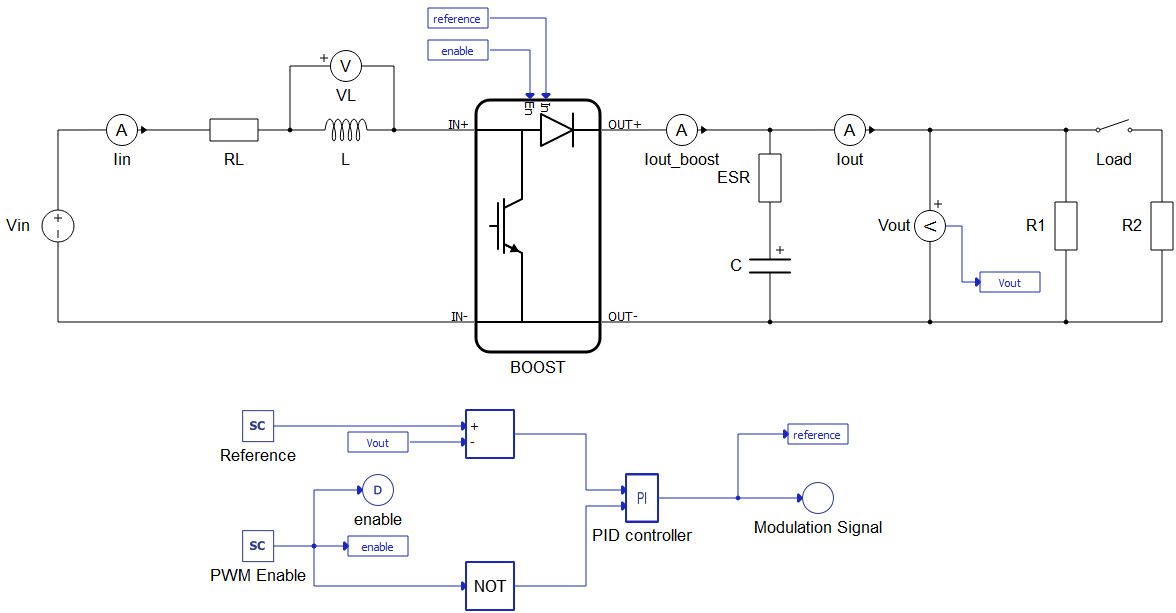# Software-in-the-loop approach in rapid control prototyping

Demonstration of two RCP implementations of a PI Controller using Schematic Editor libraries and C code import from Simulink

## Introduction

The Software-in-the-loop (SIL) concept enables the initial simulation and validation of virtual controllers. It proves the concept of the controller under development without the cost and complexity associated with testing real controllers. Rapid Control Prototyping (RCP) is a process that lets engineers quickly test and iterate their control strategies. RCP decreases development time by allowing corrections to be made early in the product process. By giving engineering a look at the product early in the design process, mistakes can be corrected and changes can be made while they are still inexpensive.

By using a RCP approach, it is possible to test and evaluate the behavior of new equipment in the early phase of the project. This example shows how SIL can enable RCP by testing and validating control of a Boost Converter.

## Model description

The electrical model of a boost convertor consists of a voltage source, an inductor with internal resistance, a capacitor with internal resistance, two types of loads, and a switch. Initial input voltage is 30 V and output voltage is 50 V. The carrier frequency of the PWM modulator is 10 kHz. The controller is implemented in two different ways: first by using Signal processing components as shown in Figure 1, and second by using the Advanced C function as shown in Figure 2. The Advanced C function in this case uses C code generated from Simulink. A tutorial on how to generate C a code file from Simulink can be found here: Software Spotlight - Integrate Simulink Model into Typhoon HIL environment.Figure 1. Model of boost converter with controller implemented using signal processing

After generating C code files from Simulink, the location of the directory should be included in the “Additional Sources” tab. The imported directory can be confirmed in the “Name” tab.

To include “Controller” functions in Schematic Editor, we use a simple output_fcn function. In output_fcn() below, you can see how to call this function.

output_fnc(){
rtU.Vout = Vout_in;
rtU.Reference = Reference_in;
rtU.PWM_Enable = PWM_Enable_in;

boost_closed_loop_control_step();

Enable_out = rtY.enable;
Modulation_Signal_out = rtY.Modulation_Signal;
}

The PI regulator is implemented by a void function “boost_closed_loop_control_step”. Inputs for this function are reference voltage, which comes from the SCADA Input component (Reference_in), and output voltage (Vout_in). This function outputs the settings for the duty cycle for the PWM modulator.

## Simulation

In this application note we can see the behavior of the PI controller. Also, we can compare the controller from Simulink and the controller implemented using the Signal Processing library. The figures below show how the controller reacts during a simple transition to the "On" state. We can see that there is no difference between these two approaches. Output power in both scenarios is 120 W.

Table 1. Minimum requirements
Files
Typhoon HIL files

examples\models\general power electronics\boost closed loop

boost closed loop.tse,

boost closed loop.cus

examples\models\how-to examples\boost closed loop advanced c